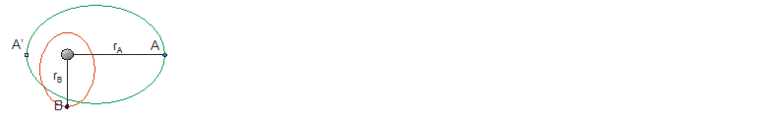# Problem: The orbits of two planets orbiting a star are shown. The semimajor axis of planet A is twice that of planet B. If the period of planet B is TB, the period of planet A is A. 2√2TB B. 3TB C. √3TB D. 4TB E. 2TB

82% (69 ratings)
###### Problem Details

The orbits of two planets orbiting a star are shown. The semimajor axis of planet A is twice that of planet B. If the period of planet B is TB, the period of planet A is

A. 2√2TB

B. 3TB

C. √3TB

D. 4TB

E. 2TBFrequently Asked Questions

What scientific concept do you need to know in order to solve this problem?

Our tutors have indicated that to solve this problem you will need to apply the Kepler's Third Law in Elliptical Orbits concept. You can view video lessons to learn Kepler's Third Law in Elliptical Orbits. Or if you need more Kepler's Third Law in Elliptical Orbits practice, you can also practice Kepler's Third Law in Elliptical Orbits practice problems.

How long does this problem take to solve?

Our expert Physics tutor, Jeffery took 2 minutes and 21 seconds to solve this problem. You can follow their steps in the video explanation above.